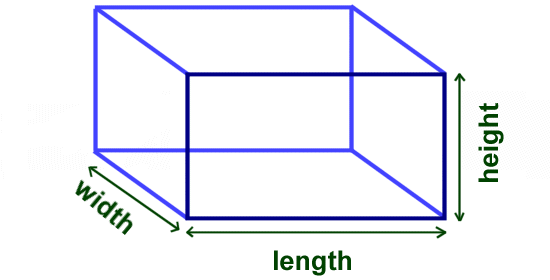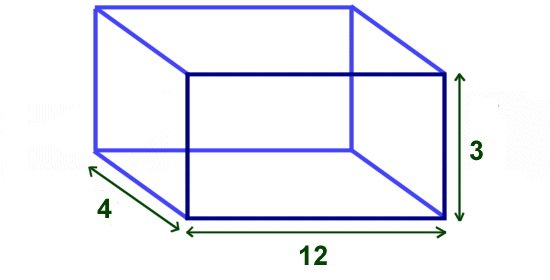Parents and Teachers: Support Ducksters by following us onor.

Kids Math

Finding the Volume of a Cube or Box

What is volume?

Volume is the measurement of how much space a three dimensional object takes up. On this page we will look at how to figure out the volume of a box.

Volume of a Box

If you know how to multiply you can find the volume of a cube or box. We learned earlier that the surface area of a flat rectangle was the length times the width, but that was just a flat two-dimensional object.

Look at the box drawing below:You see that when dealing with a box, or three dimensional object, we have to consider three measurements: length, width, and height. The formula for finding the volume is length x width x height:

l x w x h

It doesn't matter what order you multiply these together. You will get the same answer regardless of the order.

Also, the terms length, width, and height are just words to help you remember the formula. It doesn't matter which side is which. You can call the sides anything you like as long as you get the measurement for each of the three dimensions.

Example Problem

Find the volume of the box below:Here we see that

Length = 12
Width = 4
Height = 3

Volume = length x width x height

Volume = 12 x 4 x 3 = 144

The Cube

A special case for a box is a cube. This is when all the sides are the same length. You can find the volume of a cube by just knowing the measurement of one side.

If a cube has side length "a" then

Volume = a x a x a
Volume = a3

This is where we get the term "cubed". When we have something to the power of 3, we call it cubed.

Units of Measure

When you find the volume of an object, the units are cubed. This means that if the measurements of the sides were in inches, then the answer is in inches cubed or inches3.

Example:

Find the volume of a box with the following dimensions:

Length = 7 cm
Width = 2 cm
Height = 3 cm

7 x 2 x 3 = 42 cm3

Things to Remember
• Volume = length x width x height
• You only need to know one side to figure out the volume of a cube.
• The units of measure for volume are cubic units.
• Volume is in three-dimensions.
• You can multiply the sides in any order.
• Which side you call length, width, or height doesn't matter.

More Geometry Subjects

Circle
Polygons
Triangles
Pythagorean Theorem
Perimeter
Slope
Surface Area
Volume of a Box or Cube
Volume and Surface Area of a Sphere
Volume and Surface Area of a Cylinder
Volume and Surface Area of a Cone
Angles glossary
Figures and Shapes glossary

Back to Kids Math

Back to Kids StudyFollow us onor